# SQL Where: The Complete Guide

The WHERE clause in SQL extracts only those records that fulfill the specified condition. The WHERE clause is not only used in the SELECT statement; it is also used in an UPDATE, DELETE statement.

## SQL Where

SQL WHERE clause filters the database records and specifies a condition while retrieving the data from a single table or joining multiple tables. The given condition only returns a specific value from the table if the given condition is met.

If the given condition is satisfied, it only returns the specific value from the table. Therefore, you should use a WHERE clause to filter and fetch the necessary records.

The syntax of a SELECT statement with a WHERE clause is the following.

```SELECT column1, column2, columnN
FROM table_name
WHERE [condition]```

“SELECT column1, column2, column3 FROM tableName” is the standard SELECT statement.

“WHERE” is the keyword that restricts the select query result set and “condition” is a filter to be applied to the results. The filter can be the range, single value, or subquery.

Now, we will head for the example, but before that, you might check out my Create table in SQL and How to insert data in database posts.

If you have followed that tutorial, then in that tutorial, I have created a table and inserted some records.

Now, we fetch the records based on the WHERE clause.

Okay, now, first, let’s fetch all records.

For that, I need to write the following query.

`SELECT * FROM Apps`

See the following output.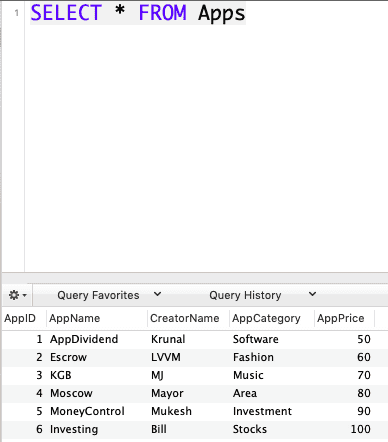SQL requires single quotes around text values. However, the numeric fields should not be enclosed in the quotes.

Okay, now let’s use the WHERE query to filter the record. But, first, let’s fetch the record by the CreatorName column.

Write the following query.

```Select * from Apps
Where CreatorName = 'Krunal'```

See the output.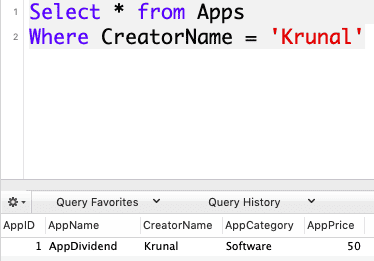Let’s fetch the row by filtering AppCategory.

```Select * from Apps
Where AppCategory = 'Investment'```

See the output.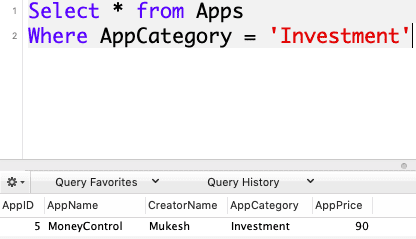You can specify the condition using the comparison or logical operators like >, <, =, LIKE, NOT, etc.

## SQL Where Statement with Logical Operator

We can use the Logical operator with the Where clause to filter out the data by comparing. For example, let’s say we fetch the data whose AppPrice is greater than 70\$.

See the following query.

```Select * from Apps
Where AppPrice > 70```

See the output.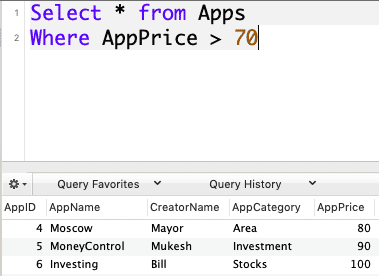## Operators in WHERE Clause

The following operators can be used in a WHERE clause.

Operator Description
= Equal
> Greater than
< Less than
>= Greater than or equal
<= Less than or equal
<> Not equal. Note: In some versions of SQL, this operator may be written as !=
BETWEEN Between the certain range
LIKE Search for the pattern
IN To specify multiple possible values for a column

### WHERE clause combined with AND LOGICAL Operator

The WHERE clause, when used with the AND operator, is only executed if All the filter criteria specified are met. See the following query.

```Select CreatorName from Apps
Where AppPrice > 70 AND AppName = 'Moneycontrol'```

So, we are fetching only CreatorName in which the AppPrice is > 70 and AppName = MoneyControl. See the below output.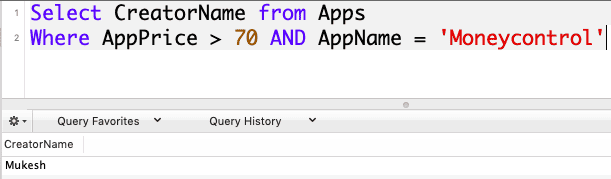### WHERE clause combined with IN Keyword

The WHERE clause, when used together with an IN keyword, only affects the rows whose values match the list of values provided in the IN keyword. IN helps to reduce the number of OR clauses you may have to use. See the following query.

```Select * from Apps
Where AppPrice IN (50, 60, 110)```

In the above query, we are fetching those records whose AppPrice is either 50, 60, or 110. If AppPrice matches in the database, it will give us that row in the output.

In our case, only 50 and 60 matches and 110 are not in the database. So, two rows will be returned.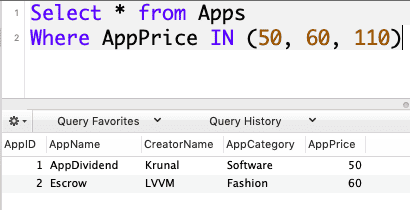### WHERE clause combined with OR LOGICAL Operator

The WHERE clause, when used with the OR operator, is only executed if any or the entire specified filter criteria are met. See the following query.
```Select * from Apps
Where AppPrice = 50 OR AppCategory = 'Fashion'```

In the above query, AppPrice = 50 holds or AppCategory = Fashion holds.

If both are true, then also it gives the result. See the output.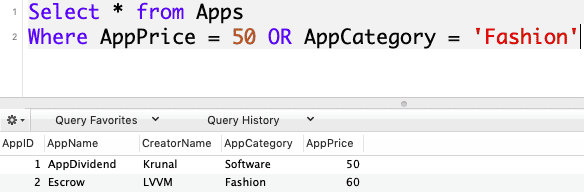### WHERE clause combined with NOT IN Keyword

The  WHERE clause used with the NOT IN keyword does not affect the rows whose values match the list of values provided in the NOT IN keyword. See the following query.

```Select * from Apps
Where AppPrice NOT IN(40, 50, 60)```

So, it will return those rows which have not AppPrice 40, 50, or 60. Then, it will give us the remaining rows.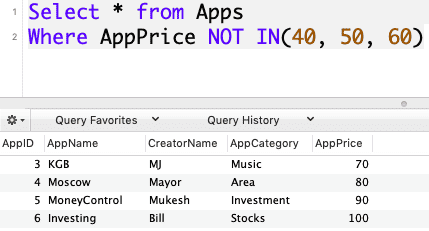The SQL WHERE statement restricts the number of rows affected by the SELECT, UPDATE or DELETE query. The WHERE term can be used with logical operators such as AND and OR, comparisons such as equals to(=), etc.

All criteria must be met when used with the AND logical operator. When used with the OR logical operator, any criteria must be met. The keyword IN is used to select rows matching the list of values.

That’s it for this tutorial.

Categories SQL

This site uses Akismet to reduce spam. Learn how your comment data is processed.